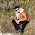## Sunday, May 16, 2010

### Irodov Problem 3.38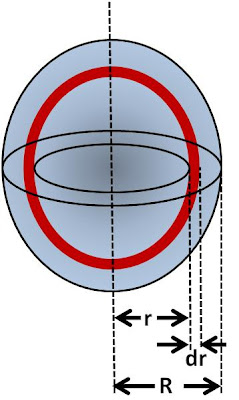a) Consider an infinitesimally thin spherical shell of thickness dr and radius r concentric with the charged sphere. The volume of this spherical shell will be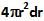. The charge density in the sphere is given by,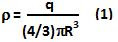Hence the charge contained in this infinitesimally thin spherical shell is given by,The electric potential due to this shell at the center of the sphere will then be,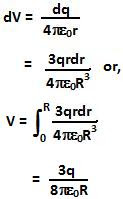b)

As derived in Problem 3.28 Eqn 2a, the electric field inside the sphere is given by,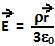The potential difference between a point that is at a distance r from the center within the sphere and the center can then be computed as,Thus the potential at a distance r from the center is given by,1.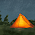2.